# 3D Rotations in Processing (Vectors, Matrices, Quaternions)

This tutorial introduces how to rotate objects in 3D beyond Euler angles; to do this, it looks at the basics of matrices and quaternions. What follows is math heavy, so a robust artistic imagination will be valuable once we dig in.

• Processing version 3.3.6 was used for the example code. A warning for PC users: In the past, working in 3D with Processing caused significant lag.
• For those who are looking for a library that has already implemented these concepts, a few are glMatrix (JavaScript), Physically Based Rendering (C++), Three (JavaScript), and Toxiclibs (Processing). They have served as references for the code that follows.

# Rotations of a Vector on X, Y and Z Axes

Suppose we’ve already got a vector, maybe constructed with Cartesian coordinates or retrieved from a `PShape`. There are no axis-specific rotations for 3D vectors. There are general rotateX and rotateY functions, which we typically place between pushMatrix and popMatrix (more on those later)… there are also PShape and PMatrix3D versions… there’s a `rotate` for 2D vectors… a rotation can be applied to a vector with `PMatrix3D`’s mult. If those suffice, this section can be skipped.

# Rotations of a Vector by Axis and Angle

1. find the elevation of the point, the component perpendicular to the axis;
2. find the plane of rotation about the axis;
3. extend a point from the plane of rotation onto the plane containing the original point.

## Project The Point Onto The Axis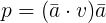The projection of point v equals the dot product of v and axis a multiplied by a.

## Find the Elevation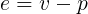The elevation of point v equals v minus its projection onto axis a.

## Find the Plane of Rotation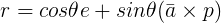The plane of rotation r equals cosine theta multiplied by the elevation plus sine theta multiplied by the axis a crossed with projection p.

## Extend a Rotated Point to the Original’s Plane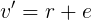The rotation of v around axis a equals the rotation r plus the elevation.

# 4 x 4 Matrices

## Looking Toward A Point

Two key interactions between vectors and matrices are multiplication (already in the `PMatrix3D` class) and the orientation of one shape to look at another. We’ll place these in the `Vec3` class.

# Quaternions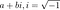A complex number is a real part, a, added to an imaginary part, b.Hamilton’s discovery.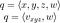A quaternion can be described with four components, x, y, z and w; or as an imaginary vector v and a real part w.

## Converting from Axis-Angles, Applying To Points

While the above gloss may keep us from misunderstanding quaternions, it doesn’t help us with how to use them. The formula to create a quaternion from an axis-angle,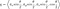A quaternion’s imaginary parts are the normalized axis a multiplied by sine half theta. Its real part is cosine half theta.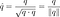A unit quaternion is normalized by dividing the quaternion by its magnitude, or the square-root of its dot product with itself.

## Easing

We can now synthesize what we learned from rotating by an amount in 3D with what we learned rotating from one angle to another in 2D.

## Converting To and From Matrices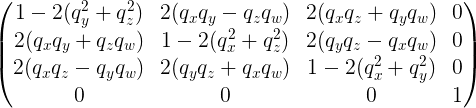Conversion from a quaternion to a matrix.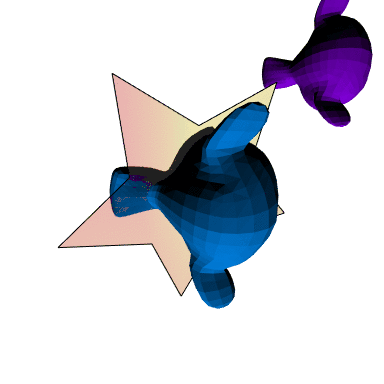A quaternion rotation applied to an immediate shape (star), retained shape (blue Suzanne), tested against a control shape (purple Suzanne).

# Conclusion

Now that some basics of rotation are taken care of, what’s available to us? This enables us to create `Transforms` which track rotation data for 3D objects in our sketch without need of `pushMatrix` and `popMatrix`. If we add Euler angle conversions to our code, we could import and interpret a BioVision Hierarchy (.bvh) file then pass it on to either matrices or quaternions. And even more opportunities open up should we choose to work more directly with OpenGL.

Creative coder from Wisconsin, USA.

## More from Jeremy Behreandt

Creative coder from Wisconsin, USA.